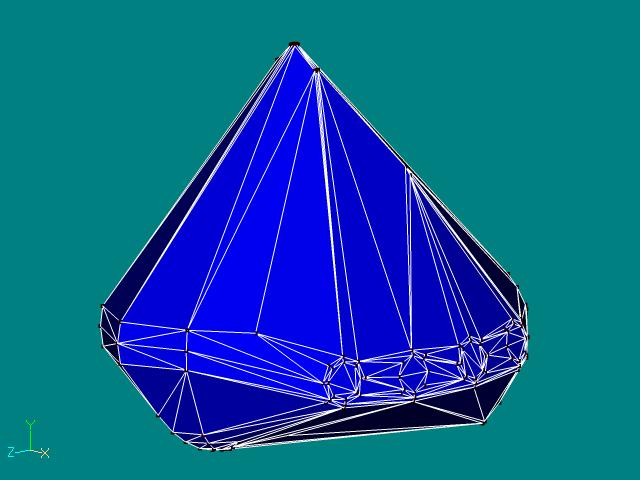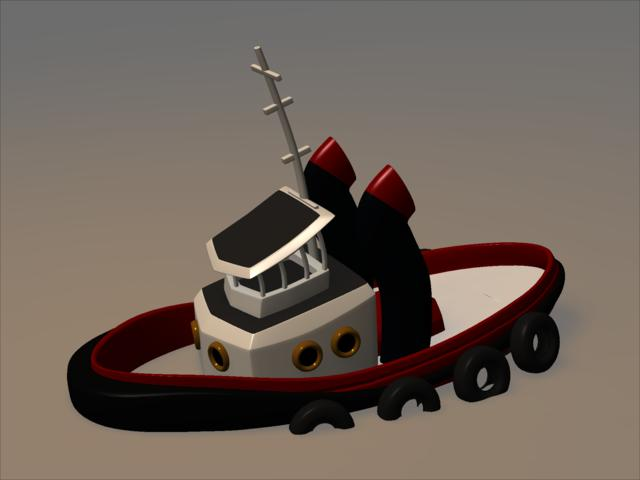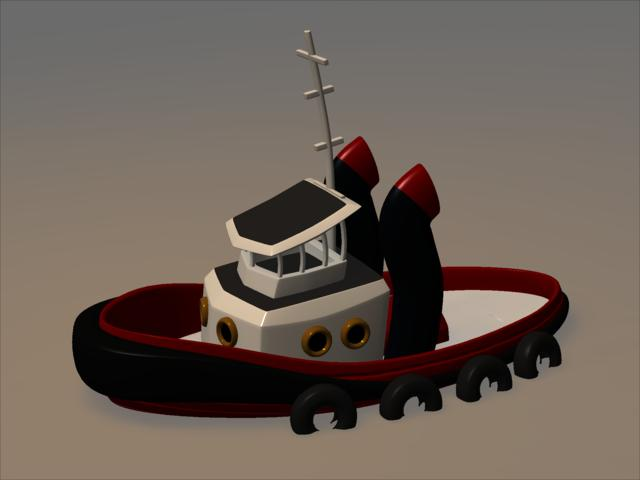# How to make the boat stable

While I'm thinking that you will run into this problem , when simulating fluids with the plugin , I would share my experience about this .In this example I use the Tugboat (the whole Projectfile can you download here), which you can find on your A:M cd . After you have added a fluidsurface and the Tugboat to a choreographie , you should set the propertie "Write logfile" to "On", set also the propertie "Use chortimerange" to "Off" , and for the propertie "End" 25 (frames) .
Save the project and simulate this the first time . What you will see , is that the boat will sink . Why this behavior , simple a convex hull around the whole model is build like this pictureand while the volume (have a look in the logfile) is around 4125 cubic meter with a density from 1 kg/cubic decimeter the mass is now 4125 tons . Offcourse this mass is to high .A ship is not a solid body :-), and because the expenditure to make the Tugboat to a nonsolid body is to high , we can simple tweak the density . Now we come to some simple mathematics (I have rounded all values up for simplification)
First the Archimedes principle with state: The force asserted over body immersed in a fluid is equal to the weight of the fluid displaced by the body and point of the direction opposite to the gravity.
Now some definitions
mass = volume * density
weightforce = gravity * mass
liftforce = volume * fluiddensity * gravity

If the liftforce is smaller than the weightforce the body will sink
If the liftforce is equal the weightforce the body is in balance
If the liftforce is greater than the weightforce the body will ascend

In our case is the weightforce 40458,69, but the liftforce is 39981,27 , so the body will sink . To get the body in balance we can easyly compute the needed density for the Tugboat with the formula
density = liftforce / (gravity*volume) now we get a density from 0,9882 (who has thinked that :-)), but with this value the boat will ever sink , while the liftforce is equal the weigthforce first when the whole body is in the fluid (you can test it , when you set the "End" for the simulation to 500 frames and resimulate , the body will stay , when it's full in the fluid .
So we had to lower the density for the boat again , and now we must estimate , we cannot compute correct the volume that is in the fluid . The boat should be at araound 25 percent into the fluid , also we count density = liftforce*0.25 / (gravity*volume) now we get for density 0.247 , now we can resimulate with this value as density for the Tugboat .
Ok , the ship does not sink longer , but it will now stip with nose into the fluid like the next picture (click on it to watch the movie)This behavior is depended to the center of mass for the collision hull , they is to much in the z+ axis from the model . Solving this is easy but needs sometimes experiments . For this example select (in the sideview) all cp's from the model and move this group 200cm in the z+ axis . Then translate the model in the chor in z- 200cm to set it return to his old place . Set the "End" time for the simulation to 250 frames and simulate again . Now it should look like the next picture (click on it to watch the movie)It sinks at the beginning a little bit , but then it swims . Problem solved :-)

Back